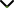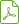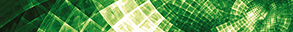# Recent Trends in Computational and Theoretical Aspects in Differential and Difference Equations

Status
Published

Guest Editors

1Indian Institute of Technology Roorkee, Roorkee, India

2Ondokuz Mayis University, Samsun, Turkey

3Abu Dhabi University, Abu Dhabi, UAE

# Recent Trends in Computational and Theoretical Aspects in Differential and Difference Equations

## DescriptionDifferential and difference equations are used for modeling, solving, and discussing many problems arising in engineering and natural sciences. Therefore, analysis of analytical and numerical solutions to such equations is crucial for applications. It is important to develop new theories and methods and to modify the well-known techniques.

The main purpose of this special issue is to attract high-quality contributions and highlight significant recent developments on the subject of differential and difference equations. The focus of this special issue is also originated with numerical methods for solving differential equations and modelling and/or considering engineering problems involving differential and difference equations. We are particularly interested in industrial mathematics problems that come from industrial and/or engineering mathematics, which take place regularly at universities across the world. These mathematical models are then solved (usually after some simplification) and conclusions relevant to the real-world problem are made. Then, these applied problems give rise to importance of theory of differential and difference equations. Original contributions that are not yet published or that are not currently under review by other journals or anywhere are sought. We will solicit high-quality original research articles as well as review articles addressing recent advances on these equations. We welcome theoretical, numerical, and experimental contributions.

Potential topics include but are not limited to the following:

• Ordinary differential equations
• Partial differential equations
• Fractional differential equations
• Stochastic differential equations
• Singular differential equations
• Delay differential equations
• Integral equations
• Difference equations
• Dynamics equations on time scale
• Finite difference methods
• Functional differential equations
• Abstract differential equations
• Mathematical modelling of engineering problems
• Stability and oscillation
• Boundary and initial value problemsDownload Special IssueJournal metrics
Acceptance rate22%
Submission to final decision43 days
Acceptance to publication32 days
CiteScore0.500
Journal Citation Indicator0.690
Impact Factor0.971Author guidelinesEditorial boardDatabases and indexing

Article of the Year Award: Outstanding research contributions of 2020, as selected by our Chief Editors. Read the winning articles.